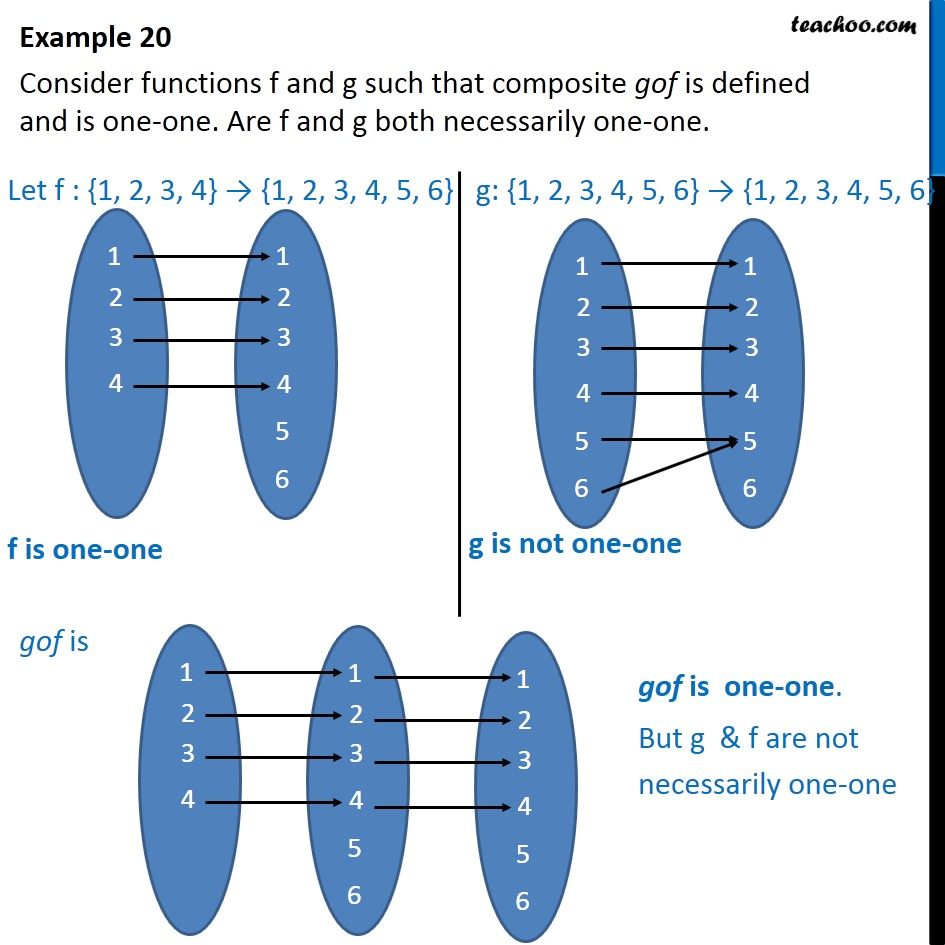1. Chapter 1 Class 12 Relation and Functions
2. Serial order wise
3. Examples

Transcript

Example 20 Consider functions f and g such that composite gof is defined and is one-one. Are f and g both necessarily one-one. Here, we take examples and function f, g And draw their set using arrow diagram Here, f is one-one But g is not one And finding gof using arrow diagram, we see that gof is one-one But g & f are not necessarily one-one

Examples